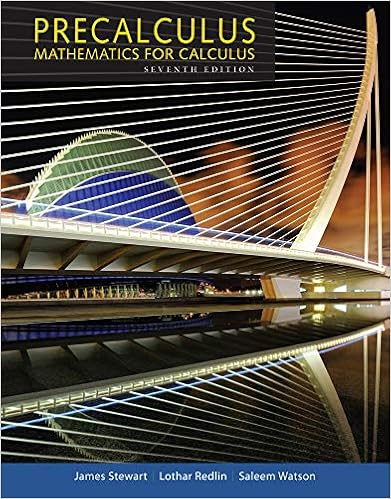# UFCalcSet10 - Exercises UF Calculus Set 10 1 Sketch a...

• Notes
• 2

This preview shows page 1 - 2 out of 2 pages.

##### We have textbook solutions for you!
The document you are viewing contains questions related to this textbook.The document you are viewing contains questions related to this textbook.
Chapter 3 / Exercise 68
Precalculus: Mathematics for Calculus
Redlin/StewartExpert Verified
ExercisesUF Calculus Set 101. Sketch a continuousfunctionfsuch that:f(1) = 4;limx→∞f(x) = 2;limx→-∞f(x) =-2.2. Evaluate the limits at infinity:
3. Write all horizontal and vertical asymptotes for the functions; list any removable discontinu-ities (holes).
(b)f(x) =x
4. Evaluate the limits at infinity in each direction for the functions:(a)y= 1-x2(b)y=x3-x(c)y=x+1x(d)y=x3/2(2-5x)(e)y=xln|x|5. Evaluate the limits at infinity in each direction using squeeze theorem(a)f(x) =sin(x)x(b)f(x) =x+ sin(x)x+ 1(c)f(x) =xesin(x)x2+ 1
##### We have textbook solutions for you!
The document you are viewing contains questions related to this textbook.The document you are viewing contains questions related to this textbook.
Chapter 3 / Exercise 68
Precalculus: Mathematics for Calculus
Redlin/StewartExpert Verified
6. Some applications are given below. Find the limit toward infinity in each case and interpretits importance.(a) When a mass on a spring is pulled back and released in the presence of friction, its
•••